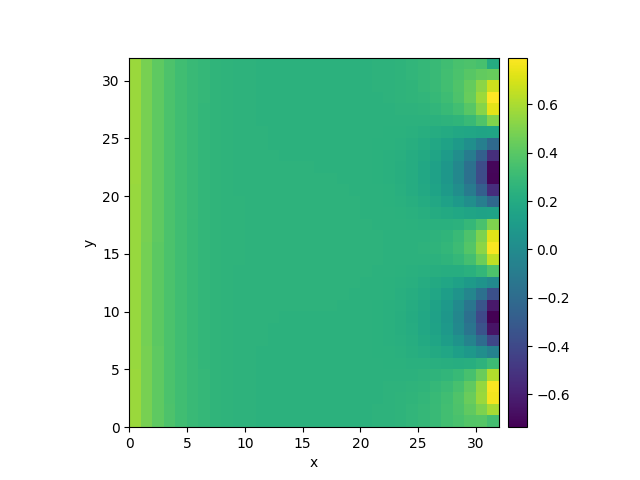# 2.11. Setting boundary conditions

This example shows how different boundary conditions can be specified.```  0%|          | 0/10.0 [00:00<?, ?it/s]
Initializing:   0%|          | 0/10.0 [00:00<?, ?it/s]
0%|          | 0/10.0 [00:05<?, ?it/s]
0%|          | 0.005/10.0 [00:05<3:05:22, 1112.85s/it]
0%|          | 0.025/10.0 [00:05<37:00, 222.59s/it]
10%|▉         | 0.955/10.0 [00:05<00:52,  5.83s/it]
10%|▉         | 0.955/10.0 [00:05<00:52,  5.86s/it]
100%|██████████| 10.0/10.0 [00:05<00:00,  1.79it/s]
100%|██████████| 10.0/10.0 [00:05<00:00,  1.79it/s]
```

```from pde import DiffusionPDE, ScalarField, UnitGrid

grid = UnitGrid([32, 32], periodic=[False, True])  # generate grid
state = ScalarField.random_uniform(grid, 0.2, 0.3)  # generate initial condition

# set boundary conditions `bc` for all axes
bc_x_left = {"derivative": 0.1}
bc_x_right = {"value": "sin(y / 2)"}
bc_x = [bc_x_left, bc_x_right]
bc_y = "periodic"
eq = DiffusionPDE(bc=[bc_x, bc_y])

result = eq.solve(state, t_range=10, dt=0.005)
result.plot()
```

Total running time of the script: (0 minutes 5.779 seconds)